The United States of America has a total of fifty (50) states. For this particular post, we will only discuss ten of these states. The ten largest of them by ranking the most largest state by total area to the smallest one. Total Area is equivalent to Total Land Area + Total Water Area.
See the list below for the most colossal US states.

👉 Wyoming
👉 Oregon
👉 Arizona
👉 New Mexico
👉 Montana
👉 California
👉 Texas

# Top 10 USA Largest States by Total Area

👉 Number 10 - The State of Wyoming
The capital of Wyoming is Cheyenne. The state has the population of over half a million. Its total land area is 97,093 sq. mi (251,470 sq. km) and its total water area is 720 sq. mi (1,864 sq. km). Overall its total area is 97,813 sq. mi (253,335 sq. km). It is the 10th largest US state in total area.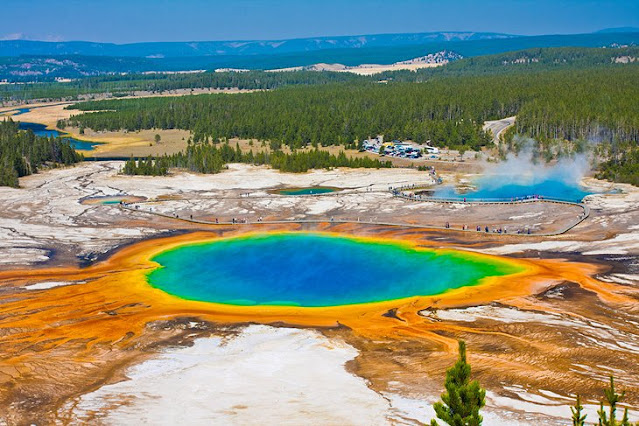Yellowstone National Park, image source: planetware

👉 Number 9 - The Oregon State
Its capital is Salem. It has a population of over 4.2 million people. Total land area is 95,988 sq. mi (248,608 sq. km) and total water area is 2,391 sq. mi. (6,191 sq. km) Combining its Total Land Area and Total Water Area, Oregon's Total Area is a staggering 98,379 sq. mi (254,799 sq. km).
👉 Number 8 - The Colorado State
Denver is its capital. It has over 5.7 million population. Total land area: 103,642 sq. mi (268,431 sq. km). Total water area is 452 sq. mi. (1,170 sq. km). Its total Area is 104,094 sq. mi (269,601 sq. km).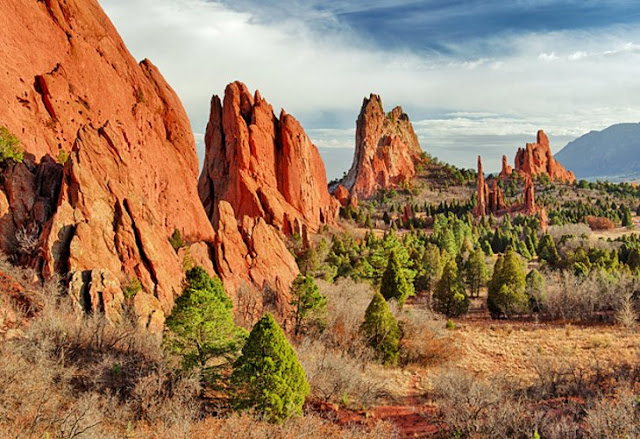Colorado Springs Garden of the gods, image source: PlanetWare

👉 Number 7 - The State of Nevada
Its capital is Carson City. It has the population of over 3.1 million. Its total land area is 109,781 sq. mi (284,332 sq. km). Its total water area is 791 sq. mi (2,048 sq. km). Total area is 110,572 sq. mi (286,380 sq. km).Las Vegas, Nevada, image source: theculturetrip

👉 Number 6 - The State of Arizona
Its capital is Phoenix and has a total population of over 7.1 million. Its total land area is 113,594 sq. mi (294,207 sq. km) and its total water area is 396 sq. mi (1,026 sq. km). Its total area summing up the total land and water areas is 113,990 sq. mi (295,234 sq. km).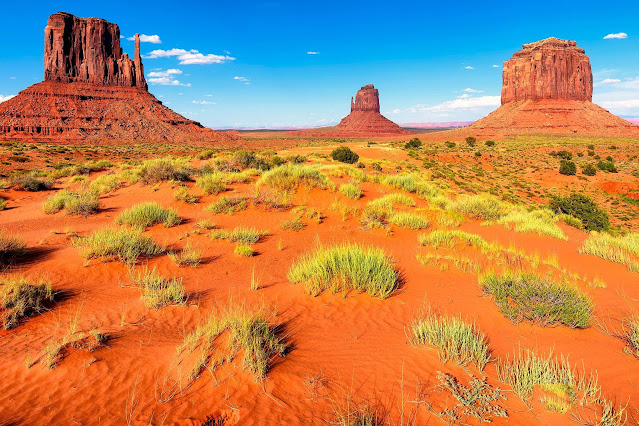Arizona Monument Valley, image source: fodors

👉 Number 5 - The State of New Mexico
New Mexico capital is Santa Fe. The state has the population of over 2.1 million people. Its total land area is 121,298 sq. mi (314,161 sq. km) and its total water area is 292 sq. mi (757 sq. km). Total area is 121,590 sq. mi (314,917 sq. km).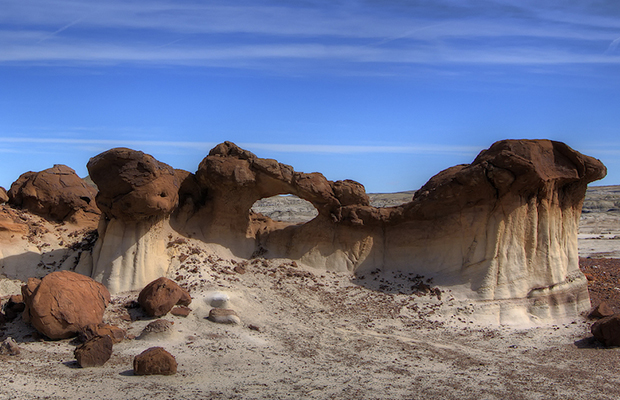Bisti Wilderness, image source: shermanstravel

👉 Number 4 - The State of Montana
Its capital is Helena and has the population of over a million. Its total land area is 145,546 sq. mi (376,962 sq. km) and its total water area is 1,494 sq. mi (3,869 sq. km). Combining the land and water areas, it has a whopping total area of 147,040 sq. mi (380,831 sq. km).Lake McDonald, image source: attractionsofamerica

👉 Number 3 - The State of California
It is the third largest state of United States in terms of total area. Its capital is Sacramento and has the population of over 39.5 million. Its total land area is 155,779 sq. mi (403,466 sq. km) and its total water area is 7,916 sq. mi (20,501 sq. mi). Total area is 163,695 sq. mi (423,967 sq. km).San Francisco Golden Gate Bridge, image source: planetware

👉 Number 2 - The State of Texas
It is the second largest state of the US. Its capital is Austin and has a population of over 29.1 million. Its total land area is 261,232 sq. mi (676,587 sq. km) and total water area of 7,365 sq. mi (19,075 sq. km). Its total are is a huge 268,596 sq. mi (695,662 sq. km).The Cross-Groom, image source: texashillcountry

👉 Number 1 - The State of Alaska
It is the largest state of the United States with a whopping total area of 665,384 sq. mi (1,723,337 sq. km). Its capital is Juneau and has a population of over 733 thousand people. Its total land area is 570,641 sq. mi (1,477,953 sq. km) and its total water area is 94,743 sq. mi (245,384 sq. km).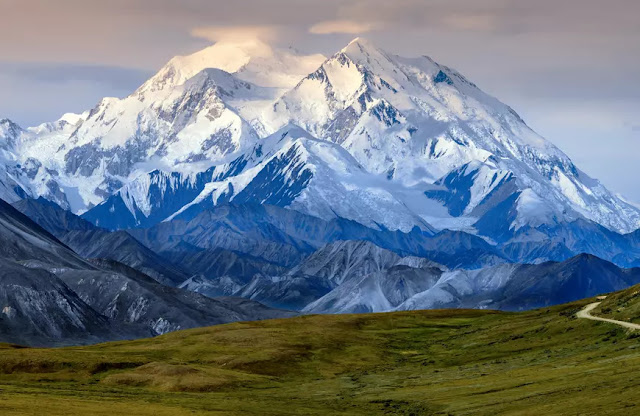Alaska's Denali, image source: thedailymeal

References:
👉 List of states and territories of the United States, Wikipedia.org
Total Page views: